# Data Science Training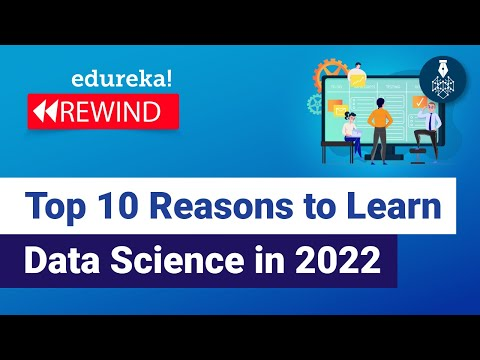Data Science

#### Top 10 Reasons to Learn Data Science in 2022

This Edureka video on “Top 10 reasons to learn Data Science in 2021” will give you enough reasons to learn data science and become a data scientist. Below are the 10 reasons covered in today’s session: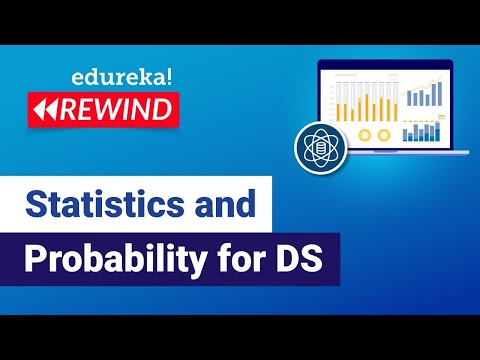Data Science Mathematics Statistics

#### Statistics and Probability for Data Science

A lot of people ask me about breaking into the fields of AI and data science. Many are surprised to hear that the core of it all revolves around statistics more so than “just code.” This session on Statistics And Probability will cover all the fundamentals of stats and probability. Topics Covered : 00:00:00 Introduction […]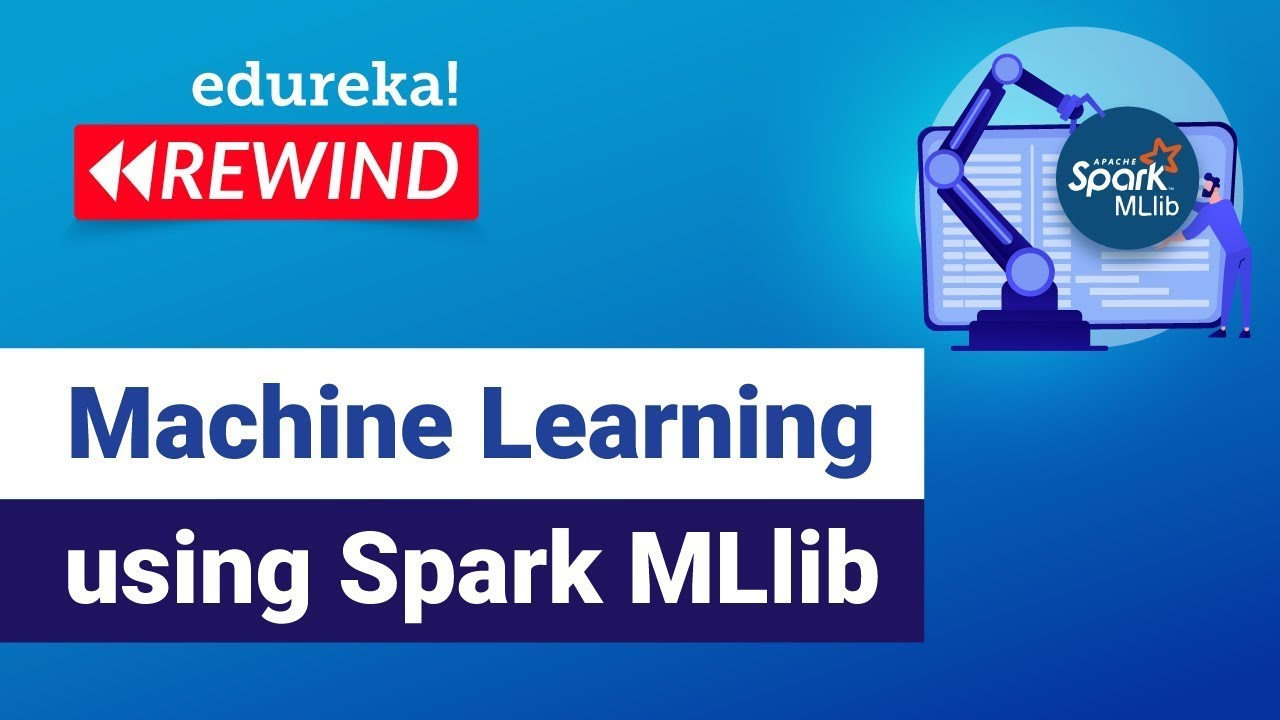Machine Learning

#### How to Get Started with Machine Learning using Spark MLlib

This Edureka “Machine Learning using Spark MLlib” video will help you to get started with Machine Learning and MLLib.Data Science

#### What is Data Science?

This Edureka video on “What is Data Science” introduces you to the concepts of Data Science and how it is used to solve real-world problems. Data science is the process of using the data to find solutions / to predict outcomes of a problem statement.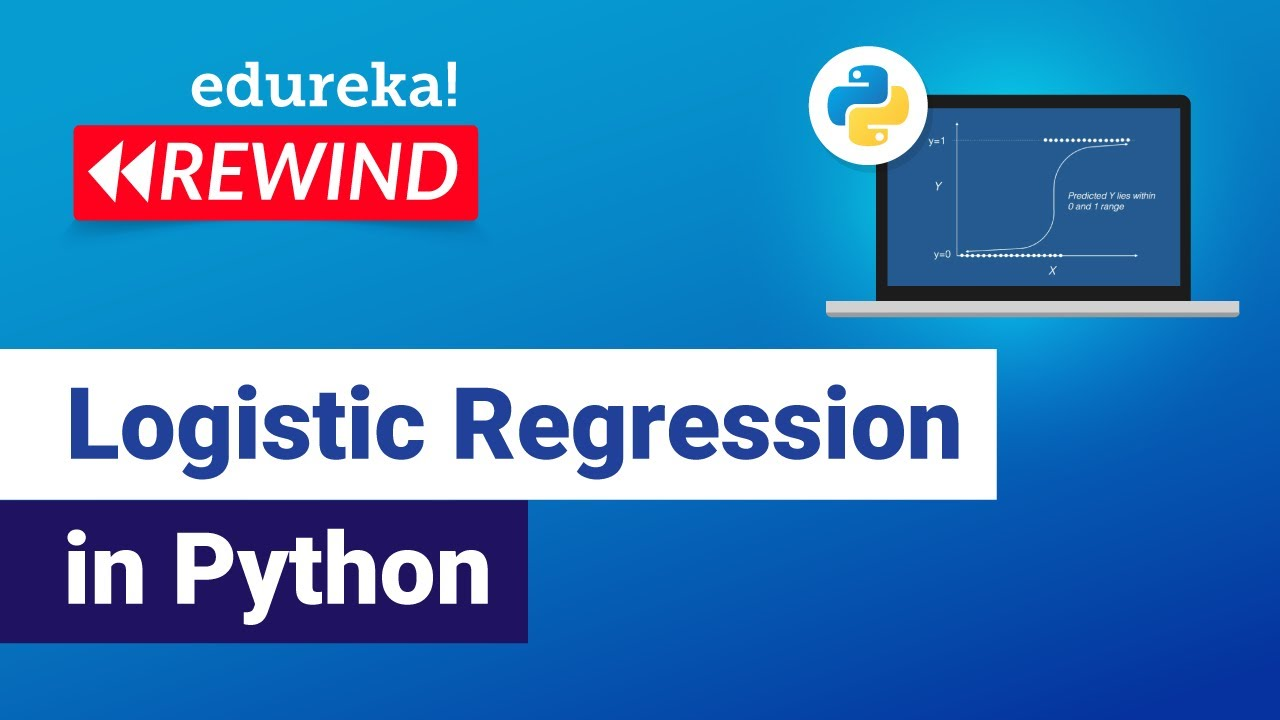Python

#### Logistic Regression in Python

This Edureka Video on Logistic Regression in Python will give you basic understanding of Logistic Regression Machine Learning Algorithm with examples. Time stamps: 00:00 Introduction 00:31 Agenda 01:13 What is Regression 03:25 Logistic Regression- What and Why 04:10 Why not Linear Regression 05:18 Logistic Regression curve 06:57 Logistic Regression Equation 08:49 Linear vs Logistic Regression […]Python

#### How to Perform Exploratory Data Analysis Using Python

Edureka shows us how to perform exploratory data analysis in Python.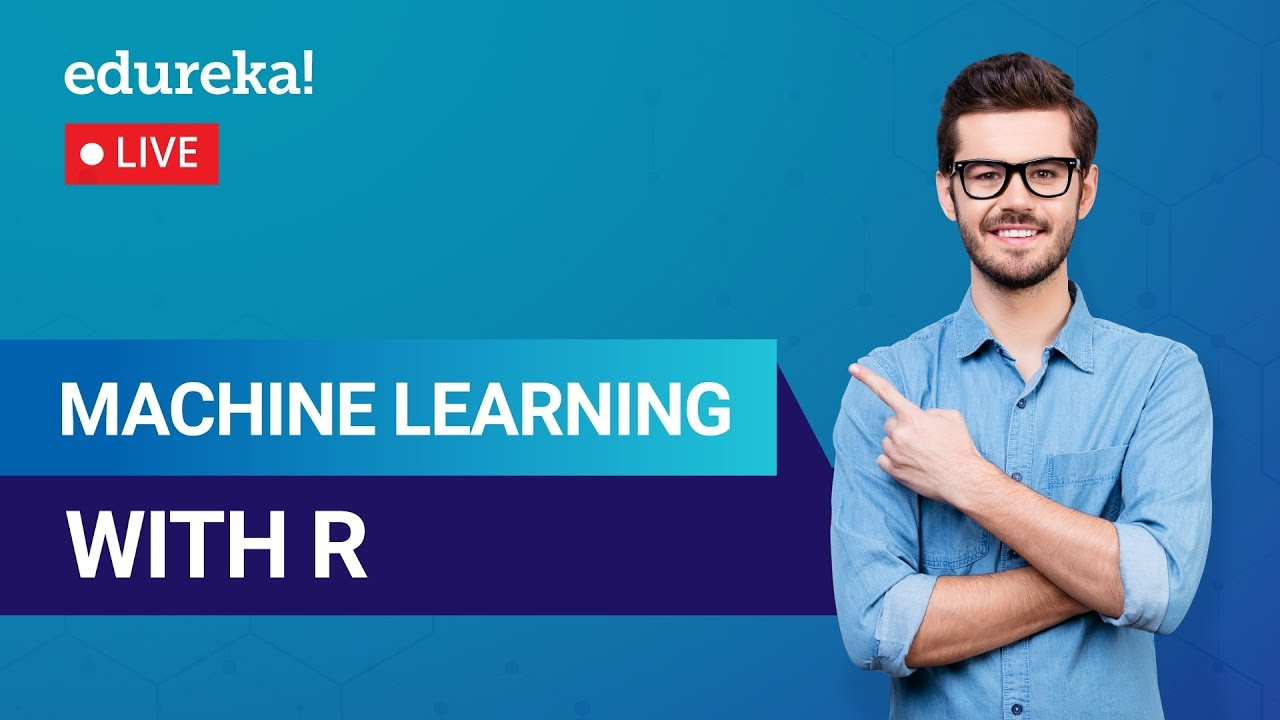Machine Learning R

#### Machine Learning with R Tutorial

edureka! provides a free tutorial on how to get started with Machine Learning in R.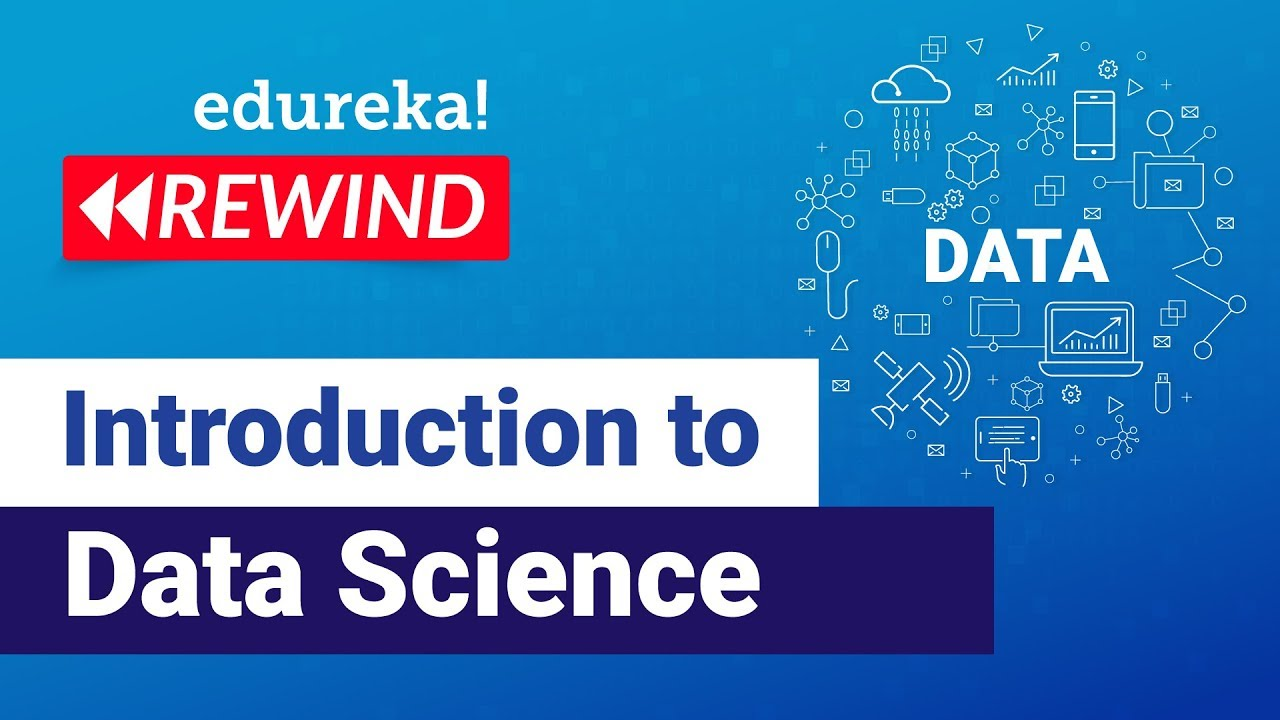Data Science

#### Introduction to Data Science

edureka! provides a basic introduction to data science in this livestream from earlier today.